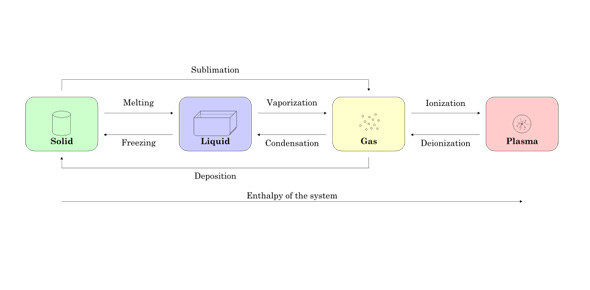# Interesting Trivia Quiz On States Of Matter

10 Questions | Total Attempts: 880SettingsStates of Matter exist since the beginning of the universe. The four states of matter are namely solid, liquid gas, and plasma. The qualitative differences differ for all states of matter. This quiz has been designed to test your knowledge about the different theories and transforming of matter into another form. Let's try this quiz and seek answers. Good Luck!

• 1.
At what temperature does water boil?
• A.

100 fahrenheit

• B.

32 celcius

• C.

103 fahrenheit

• D.

103 celcius

• E.

At the freezing point.

• 2.
At what temperature do you see mercury boil?
• A.

200 fahrenheit

• B.

500 fahrenheit

• C.

355 celcius

• D.

200 celcius

• E.

At the sublimation point

• 3.
At what temperature does methanol freeze?
• A.

99 celcius

• B.

99 fahrenheit

• C.

300 fahrenheit

• D.

-99 fahrenheit

• E.

At the melting point

• 4.
Which theory describes the arrangement and movement of particles in solids, liquids, and gases?
• A.

Theory of relativity

• B.

Kinetic theory

• C.

Atomic theory

• 5.
In which state of matter are the particles mostly touching but arranged in a random way?
• A.

Solid

• B.

Liquid

• C.

Gas

• 6.
Why can liquids not be compressed easily?
• A.

The particles are in a random arrangement

• B.

The particles are closely packed

• C.

The particles are free to move over each other

• 7.
What is sublimation?
• A.

When a solid turns into a gas

• B.

When a gas turns into a solid

• C.

When a gas turns into a liquid

• 8.
What eventually happens if energy is continually removed from a liquid?
• A.

It boils

• B.

It evaporates

• C.

It freezes

• 9.
Which of the following will sublime at room temperature and pressure?
• A.

Ice

• B.

Graphite

• C.

Solid carbon dioxide (dry ice)

• 10.
Why do solids have a fixed shape?
• A.

The particles are fixed in place

• B.

The particles are closely packed

• C.

The particles vibrate

Related TopicsBack to top